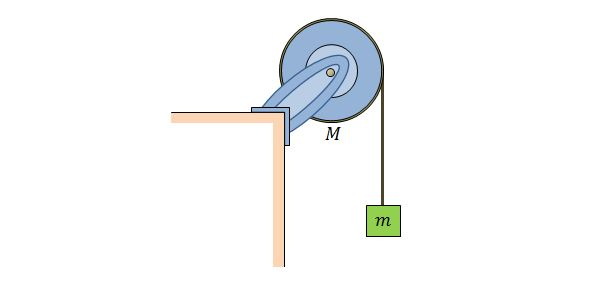Classical Mechanics

# Rotational Work-Kinetic Energy TheoremA disk is mounted on an axis, as shown in the above figure. The radius of the disk is $0.20\text{ m}$ and the axis is frictionless. A block with mass $12.0\text{ kg}$ hangs from a massless cord that is wrapped around the rim of the disk. The rotational inertia of the disk about the axis is $40.0\text{ kg}\cdot\text{m}^2$ and the system is released from rest. If the kinetic energy of the block became $12.0\text{ J},$ what is the approximate distance the block has fallen, assuming that the gravitational acceleration is $g=9.8\text{m/s}^2?$

A $1.5\text{ m}$ thin rod with mass $0.900\text{ kg}$ is standing vertically with one end on the floor. Then, if it is allowed to fall, what is the approximate speed of the other end just before it touches the floor, assuming that the gravitational acceleration is $g=9.8\text{ m/s}^2?$

Note: As shown in the figure below, the rotational inertia of a thin rod that rotates about the axis through its center perpendicular to length is $\displaystyle I=\frac{1}{12}ML^2,$ where $M$ and $L$ are the mass and length of the thin rod, respectively.A thin hoop of mass $29.0\text{ kg}$ and radius $1.2\text{ m}$ is rotating at $280\text{ rev/min}.$ If it must stop in $15.0\text{ s},$ approximately how much work must be done to it?

A uniform thin rod with length and mass of $7.40\text{ m}$ and $140\text{ kg},$ respectively, is attached to a rotational axle at its one end by a single bolt. Then how much work is needed to increase the speed of the rod to $320\text{ rev/min}$ from rest?

As shown in the figure below, the rotational inertia of a thin rod that rotates about the axis through its center perpendicular to length is $\displaystyle I=\frac{1}{12}ML^2,$ where $M$ and $L$ are the mass and length of the thin rod, respectively.A Frisbee throwing disk flies straight only if you spin it. When we spin a Frisbee we generate angular momentum, which is conserved. Since it is conserved the Frisbee resists tilting, which would change the angular momentum, and so it tends to fly straight. However, giving a Frisbee angular momentum also takes energy. If I spin a Frisbee faster and so double its angular momentum, by what factor have I increased the rotational kinetic energy?

×